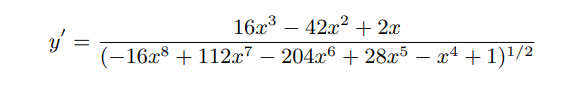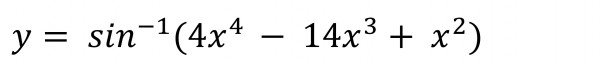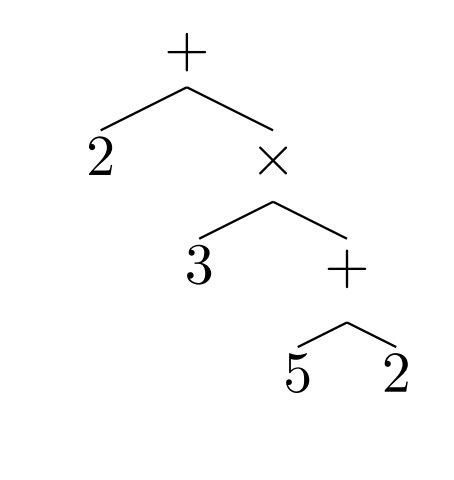# Facebook has a neural network that masters higher mathematics

17. December 2019

Facebook has a neural network that masters higher mathematics

New York, 17.12.2019

So far, neural networks have never gone beyond simple addition and multiplication. However, this calculates integrals and solves differential equations. Here is a challenge for the mathematically gifted among you. Solve the following differential equation for y:You have 30 seconds.

The answer is, of course:If you couldn’t find a solution, you don’t feel inferior. This expression is so tricky that even several powerful math software packages failed even after 30 seconds of number processing.

Guillaume Lample and François Charton of Facebook AI Research in Paris say that today they have developed an algorithm that does this at a glance. They have previously trained a neural network to perform the necessary symbolic considerations to distinguish and integrate mathematical expressions for the first time. The result is a significant step towards more powerful mathematical thinking and a new method of applying neural networks that goes beyond traditional pattern recognition tasks.

First, some background information. Neural networks have proven enormously useful in pattern recognition tasks such as face and object recognition, certain types of natural language processing, and even in games such as Chess, Go, and Space Invaders.

For neural networks and humans, one of the difficulties with advanced mathematical expressions is the abbreviation on which they rely. For example, the expression x3 is a short notation of x multiplied by x multiplied by x. In this example, “multiplication” is an abbreviation for repeated addition. This, in turn, is an abbreviation for the total value of two combined quantities.

It is easy to see that even a simple mathematical expression is a highly compressed description of a sequence of much simpler mathematical operations.

So it is no wonder that neural networks have to struggle with this kind of logic. If they do not know what the shorthand represents, there is little chance that they will learn to use it. In fact, people have a similar problem that often occurs at a young age.

At the fundamental level, however, processes such as integration and differentiation still involve pattern recognition tasks, even if they are hidden by mathematical abbreviations.

Lample and Charton, on the other hand, found an elegant way to break down mathematical shorthand into its basic units. They then train a neural network to recognize the patterns of mathematical manipulation that correspond to integration and differentiation. Finally, they let go of the neural network of expressions it has never seen before and compare the results with the answers derived from conventional solvers such as Mathematica and Matlab.

The first part of this process is to decompose mathematical expressions into their constituent parts. Lample and Charton represent expressions as tree-like structures. The leaves on these trees are numbers, constants, and variables such as x; the internal nodes are operators such as addition, multiplication, differentiation with respect to, and so on.

For example, the expression 2 + 3 x (5 + 2) can be written as :Trees are equal if they are mathematically equivalent. For example

2 + 3 = 5 = 12 – 7 = 1 x 5 are all equivalent; therefore their trees are also equivalent.

Many mathematical operations are easier to handle this way. “For example, simplifying the expression means finding a shorter equivalent representation of a tree,” say Lample and Charton.

These trees can also be written as sequences, each node being taken one after the other. In this form they are ready for processing by a neural network approach called seq2seq.

Interestingly, this approach is also often used for machine translation, where a sequence of words in one language must be translated into a sequence of words in another language. Indeed, Lample and Charton say that their approach treats mathematics essentially as a natural language.

The next step is the training process, and this requires a huge database of examples to learn from. Lample and Charton create this database by randomly compiling mathematical expressions from a library of binary operators such as addition, multiplication, etc. Unary operators such as cos, sin, and exp; and a set of variables, integers, and constants such as π and e. They also limit the number of internal nodes to prevent the equations from becoming too large.

Even with a relatively small number of nodes and mathematical components, the number of possible expressions is very large. Each random equation is then integrated and differentiated using a computer algebra system. An expression that cannot be integrated is discarded.

In this way, the researchers generate an extensive training dataset consisting of 80 million examples of first and second order differential equations and 20 million examples of partially integrated expressions.

Finally, Lample and Charton test their neural network by feeding 5,000 previously unknown expressions and comparing the results obtained in 500 cases with the results of commercially available software such as Maple, Matlab and Mathematica.

These software packages use an algorithmic approach developed in the 1960s by the American mathematician Robert Risch. Risch’s algorithm, however, is huge and contains 100 pages for integration alone. Therefore, symbolic algebra software often uses reduced versions to speed things up.

The comparisons between these and the neural network approach are revealing. “We find that our model clearly outperforms Mathematica in all tasks,” the researchers say. “In function integration, our model achieves nearly 100% accuracy, while Mathematica barely achieves 85%. And the Maple and Matlab packages perform on average worse than Mathematica.

In many cases, traditional packages can’t find a solution within 30 seconds. For comparison: The neural network needs only one second.

An interesting result is that the neural network often finds several equivalent solutions for the same problem. This is because mathematical expressions can usually be written in many different ways. This ability is an exciting puzzle for researchers. “The ability of the model to restore equivalent expressions without having been trained is very fascinating,” say Lample and Charton.

This is a major breakthrough. To the best of our knowledge, no study has investigated the ability of neural networks to recognize patterns in mathematical expressions,” the couple say, “and the result now has enormous potential in the increasingly important and complex world of computational mathematics.

The researchers do not reveal Facebook’s plans for this approach. However, it is very likely that Facebook will offer this service because it is better than that of the market leaders. Equally likely, however, is that the degraded will not sit still. Consequence: Expect a powerful battle in the world of computer mathematics.

Ref: arxiv.org/abs/1912.01412: Deep Learning for Symbolic Mathematics

Hits: 11